Pay with
Markets
Derivatives
Earn
NFT
Institutional
Feed
Cancel

# FAQAccount FunctionsTutorialBinance Fan TokenBinance EarnCrypto Deposit/Withdrawal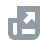Crypto Derivatives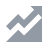FinanceAPISecurityOther Topics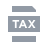Tax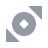Binance Convert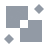NFTVIP
Home
Support Center
FAQ
Crypto Derivatives
Futures Contracts
USDⓈ-M Futures Contracts
How to Calculate Cost Required to Open a Position in Perpetual Futures Contracts

# How to Calculate Cost Required to Open a Position in Perpetual Futures Contracts

2020-07-08 06:39
Traders should ensure that they have a minimum amount of funds in their wallet balance before opening a position. The cost required to open a position includes the initial margin and open losses (if any). Open losses occur when the price of a futures contract goes unfavorably (i.e. mark price is lower than the order price for a long order). Binance includes open losses as one of the costs required to open a position to avoid forced liquidation when traders place an order. If the open loss is not included as one of the costs required to open a position, there is a high probability that users’ position will get liquidated immediately once they have placed such order.
The formula to calculate the cost required to open a position is as such:
Cost = Initial Margin + Open Loss (if any)

## Step 1: Calculate the Initial Margin

Initial Margin
= Notional Value / Leverage
= (9,253.30 * 1 BTC) / 20
= 462.66

## Step 2: Calculate Open Loss

Open Loss
= Number of Contract * Absolute Value {min[0, direction of order * (mark price - order price)]}
direction of order: 1 for long order；-1 for short order
(i) Open loss of long order
= Number of Contract * Absolute Value {min[0, direction of order * (mark price - order price)]}
= 1 * Absolute Value {min[0, 1 * (9,259.84 - 9,253.30)]}
= 1 * Absolute Value {min[0, 6.54]}
= 1 * 0
= 0
There is no open loss when the user opens a long order.
(ii) Open loss of short order
= Number of Contract * Absolute Value {min[0, direction of order x (mark price - order price)]}
= 1 * Absolute Value {min[0, -1 * (9,259.84 - 9,253.30)]}
= 1 * Absolute Value {min[0, -6.54]}
= 1 * 6.54
= 6.54
There is an open loss when the user opens a short order.

## Step 3: Calculate the cost required to open a position

Since the long order has no open loss, thus the cost required to open a long position is equivalent to the initial margin.
(i) Cost required to open a long position
= 462.66 + 0
= 462.66
Short order has an open loss, thus the cost required to open a short position is higher as we need to take open loss into consideration besides the initial margin.
(ii) Cost required to open a short position
= 462.66 + 6.54
= 469.20 (rounding difference)

## Step 1: Calculate assuming price

Long order: assuming price = ask * (1 + 0.05%) , Short order: assuming price = bid
(i) Assuming price of long order
= ask * (1 + 0.05%)
=10461.78 * (1 + 0.05%)
= 10467.01089
(ii) Assuming price of short order
= bid
= 10461.77
*: Level 1 price

## Step 2: Calculate the Initial Margin

Initial Margin = Notional Value / Leverage
(i) Initial margin of long order
= Assuming price of long order * Number of Contract / Leverage
= 10467.01089 * 0.2 / 20
= 104.6701089
(ii) Initial margin of short order
= Assuming price of short order * Number of Contract / Leverage
= 10461.77 * 0.2 / 20
= 104.6177

## Step 3: Calculate Open Loss

Open Loss
= Number of Contract * Absolute Value {min[0, direction of order * (mark price - order price)]}
direction of order: 1 for long order；-1 for short order
(i) Open loss of long order
= Number of Contract * Absolute Value {min[0, direction of order * (mark price - asuumingprice)]}
= 0.2 * Absolute Value {min[0, 1 * (10461.83 - 10467.01089)]}
= 0.2 * Absolute Value {min[0, -5.18089]}
= 0.2 * 5.18089
= 1.036178
There is an open loss when the user opens a long order.
(ii) Open loss of short order
= Number of Contract * Absolute Value {min[0, direction of order * (mark price - assumingprice)]}
= 0.2 * Absolute Value {min[0, -1 * (10461.83 - 10461.77)]}
= 0.2 * Absolute Value {min[0, 0.0599999]}
= 0.2 * 0.0599999
= 0.012
There is no open loss when the user opens a short order.

## Step 4: Calculate the cost required to open a position

Long order has an open loss, thus the cost required to open a long position is higher as we need to take open loss into consideration besides the initial margin.
(i) Cost Required to Open a Long Position
= 104.6701089 + 1.036178
= 105.71 (rounding difference)
Since the short order has no open loss, thus the cost required to open a short position is equivalent to the initial margin.
(ii) Cost Required to Open a Short Position
= 104.6178 + 0.012
= 104.63 (due to the update of assuming price calculation logic)